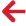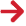﻿ PipeLay > Technical Notes > Technical Note 7 - Calculation of Moment/Curvature Curves for Pipe Elements with a Stress/Strain Material Specification > Calculation with a Tension Input

# Calculation with a Tension InputThe goal of the moment-curvature calculation procedure in PipeLay is to accurately quantify the bending moments acting on the cross-section for a set of global curvatures using a user-defined stress-strain curve. The capturing of possible tension effects on the bending moments is also an important aspect of the calculation procedure. The paragraph below provides more details on the effect of tension during pipe bending.

During installation a pipeline is likely to experience relatively high axial tension. Such tension causes a shift in the pipes neutral axis position and utilises a certain proportion of the pipes overall longitudinal stress-strain capacity before bending is considered. This in turn reduces the pipe bending capacity, or in other words for the same curvature a lower bending moment is sustained in the case of an axially loaded pipe joint than in the case of a joint with no axial load at all. Accounting for this behavior is needed for the correct estimation of stresses and strains in the pipe joint, especially when the joint is traveling along stinger supports where it is subjected to significant bending in combination with high tension. With this in mind, PipeLay provides the user with the option of defining an expected tension value along with the pipes material stress-strain curve. Note that as an alternative to specifying an expected tension value, PipeLay can be instructed to instead use the maximum value for a tension criterion, as defined on the Analysis component.

The first task for the moment-curvature calculation procedure is to determine a suitable set of global curvature values. This is done by assuming that the curvature values range from zero to a maximum value based on a maximum absolute strain at the surface of the pipe. This maximum absolute strain is obtained from the pipes stress-strain definition. The curvature range is divided in equal intervals based on the number of points in the stress-strain curve.

The algorithm that computes the associated bending moment for any given curvature must generally iterate on the displacement of the neutral axis from the pipe centreline with an aim of balancing the total axial force (inclusive of the tensile and compressive sides) with the input tensile force. The algorithm is initialised with a zero displacement for the neutral axis and the following steps are taken:

1.The tensile region of pipe cross-section is divided into equidistant slices parallel to the neutral plane, starting from the position of the neutral axis and ending at the outer boundary

2.For each of these slices, the axial force and bending moment are calculated as follows:

a.A total strain value is determined by the product of the curvature value and the arm from the neutral axis to the middle of the slice. This is then converted to a total stress by interpolation of the specified stress-strain curve.

b.The stress from the input tension is subtracted from the total stress value above to obtain a purely bending stress.

c.The total axial force in the slice is computed by scaling the total stress value from Step 2a by the slice area.

d.The area is also used to determine a slice bending force value from the bending stress provided in Step 2b. This bending force is multiplied by the arm from the neutral axis to obtain a bending moment for the slice.

3.The overall force and moment for the tensile region are calculated by summing over the individual slice forces and moments provided by Step 2.

4.Steps 1-3 are repeated in a similar fashion for the compressive region of the pipe.

5.The total axial force and bending moment for the entire pipe cross-section are calculated by adding together the forces and moments from the tensile and compressive regions of the pipe.

6.The iteration algorithm is considered to have converged if the total axial force equals the tensile force input.

a.If this is the case, then the value for the bending moment is stored and the algorithm can be repeated for a different global curvature.

b.Otherwise, an incremental displacement is applied to the neutral axis, and a new iteration is started at Step 1.

Needless to say, the iteration algorithm above is simplified when no input tension is specified. Note that the algorithm itself can be extended to successfully consider pipe sections with external coatings as well, as detailed in the next section. It is also worth highlighting that in addition to a moment-curvature curve an axial force-displacement curve is determined for elements with stress-strain input; however the calculation procedure for this curve is relatively trivial as it only requires scaling the defined stress points by the cross sectional area to give a total force value.